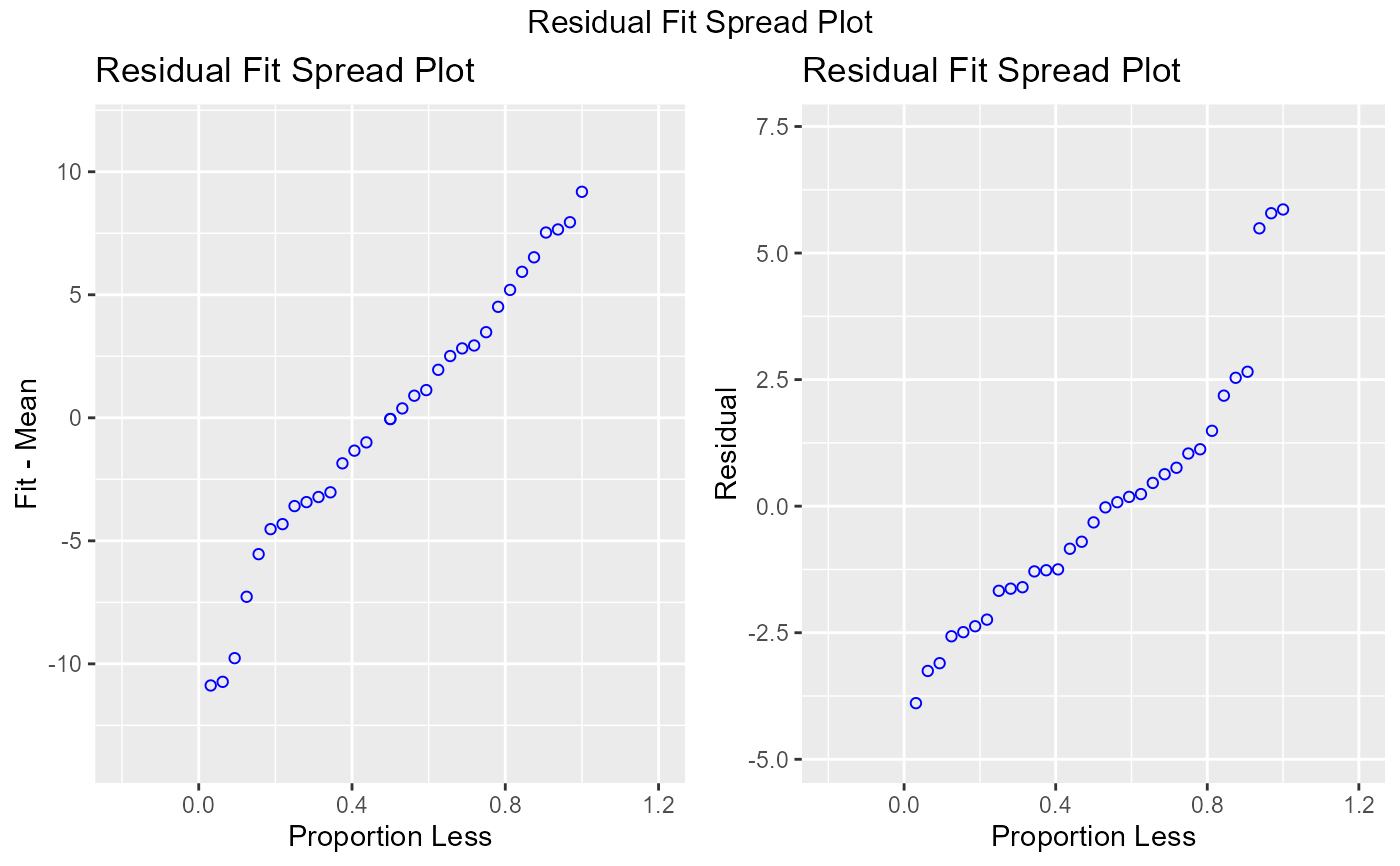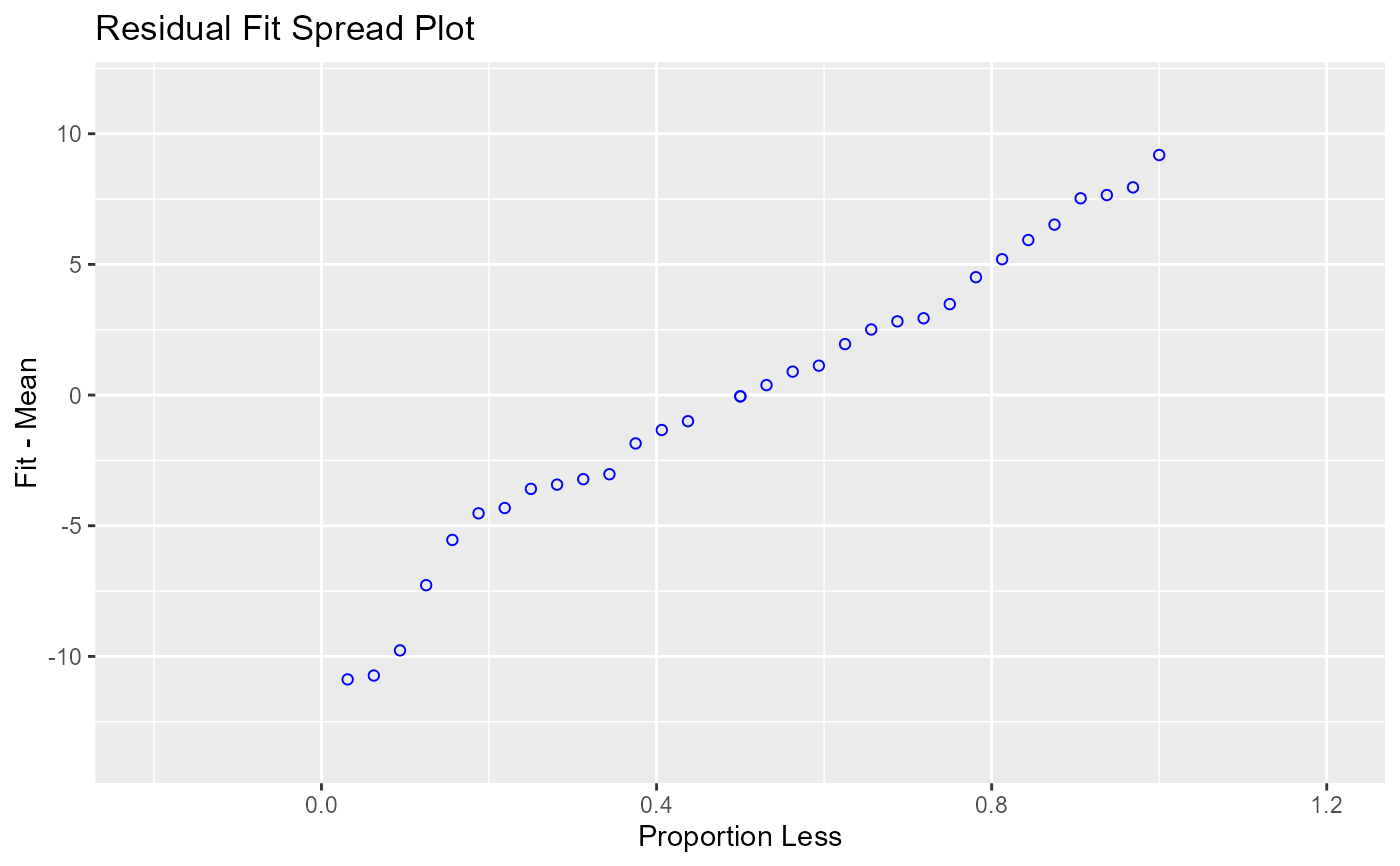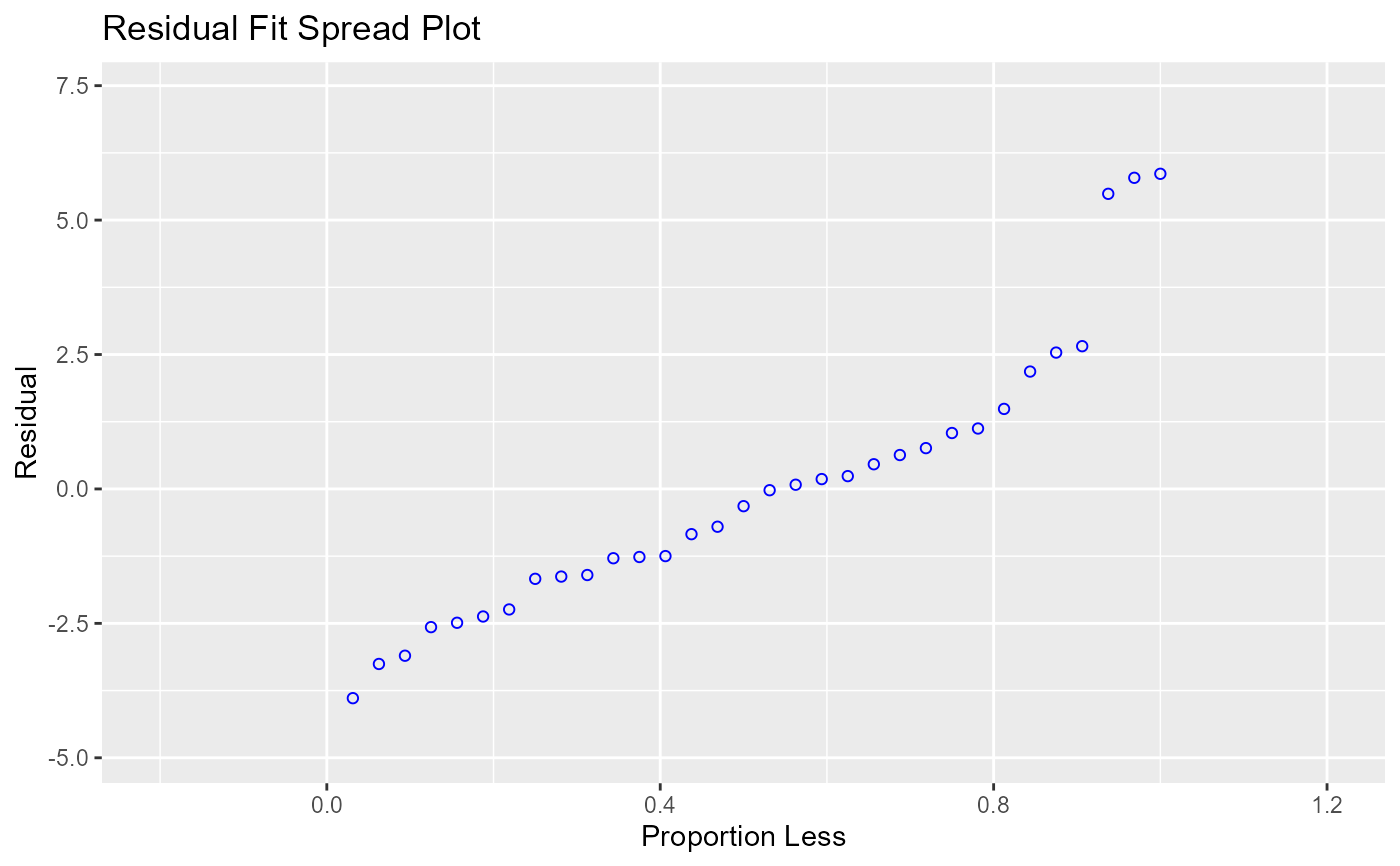Plot to detect non-linearity, influential observations and outliers.

ols_plot_resid_fit_spread(model, print_plot = TRUE)

ols_plot_fm(model, print_plot = TRUE)

ols_plot_resid_spread(model, print_plot = TRUE)

## Arguments

model An object of class lm. logical; if TRUE, prints the plot else returns a plot object.

## Details

Consists of side-by-side quantile plots of the centered fit and the residuals. It shows how much variation in the data is explained by the fit and how much remains in the residuals. For inappropriate models, the spread of the residuals in such a plot is often greater than the spread of the centered fit.

## Deprecated Function

ols_rfs_plot(), ols_fm_plot() and ols_rsd_plot() has been deprecated. Instead use ols_plot_resid_fit_spread(), ols_plot_fm() and ols_plot_resid_spread().

Cleveland, W. S. (1993). Visualizing Data. Summit, NJ: Hobart Press.

## Examples

# model
model <- lm(mpg ~ disp + hp + wt, data = mtcars)

ols_plot_resid_fit_spread(model)ols_plot_fm(model)ols_plot_resid_spread(model)